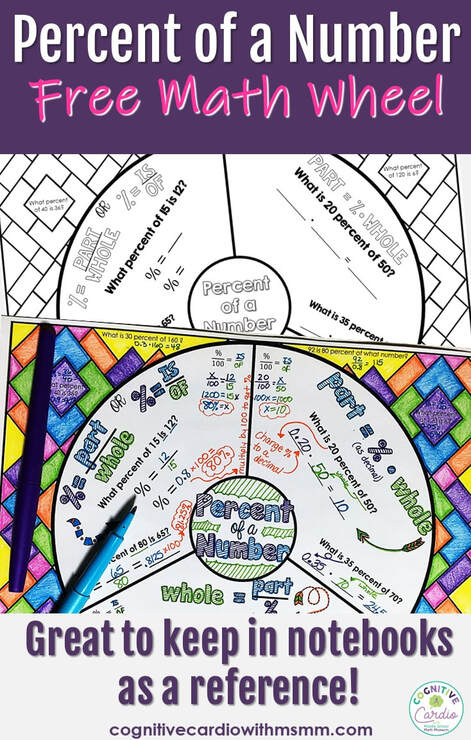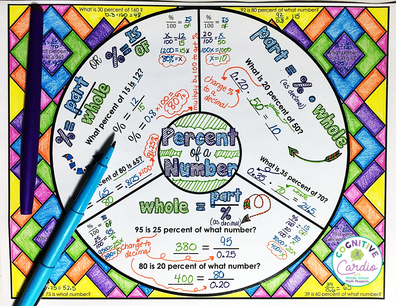# Percent of a Number Math Wheel

Teaching Percent of  Number in Middle School MathWhat method do you use for teaching percent of a number concepts in middle school math?

When I teach students to find the percent of a number (or the part or whole), I introduce two different ways to find the missing number – using proportions and using equations. Since different math students often prefer different methods, I teach both, have students practice both, and then let them choose the method they like better. I’ve given an example of each method below.

The Percent of a Number Wheel shown here includes both methods. Each section of the wheel includes an equation and two examples, with room to solve using both methods. There’s also a little room on the wheel (or around it) to add extra notes or your own examples, if you’d like. Around the wheel are a few practice problems that can be completed together or individually.

 Method 1: Proportion 1) Substitute the given values into the %/100 = IS/OF proportion. Use a variable for the missing number. 2) Solve the proportion to find the missing value. ​Example: What is 15% of 70?Click to download free percent of a number math wheel.

Method 2: Equation
1) When given the percent, change it to a decimal.
2) Substitute the given values into the equation. Use a variable for the missing number.
3) Solve the equation.
* If finding the percent, be sure the  answer is in percent form (multiply the decimal answer by 100).
Example: What is 15% of 70?
part = % ∙ whole
x = 0.15 ∙ 70
x = 10.5

When we work with the equations, I do manipulate the equations to show students how they are all versions of the same basic equation.
For example, if we start with part = % ∙ whole and we’re looking for the whole (say the part is 35 and the percent is 25), we end up with
35 = 0.25 ∙ x. From solving algebraic equations, students know that to
find x, both sides will be divided by 0.25, which gives them
x = 35/0.25
(whole = part/%)

If you decide to use the wheel, I hope you and your students like it!

If you’re looking for more percent of a number resources, check out the Percent of  a Number Center Resources on TPT.

## EllieWelcome to Cognitive Cardio Math! I’m Ellie, a wife, mom, grandma, and dog ‘mom,’ and I’ve spent just about my whole life in school! With nearly 30 years in education, I’ve taught:

• All subject areas in 4th and 5th grades
• Math, ELA, and science in 6th grade (middle school)

I’ve been creating resources for teachers since 2012 and have worked in the elearning industry for about five years as well!